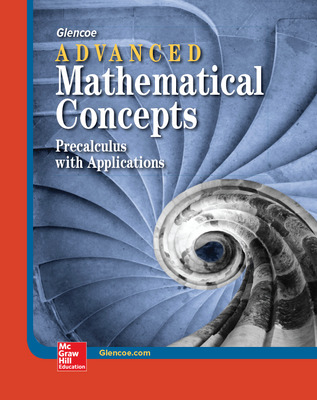# Advanced Mathematical Concepts: Precalculus with Applications

Advanced Mathematical Concepts provides comprehensive coverage of all the topics covered in a full-year Precalculus course. Its unique unit organization readily allows for semester courses in Trigonometry, Discrete Mathematics, Analytic Geometry, and Algebra and Elementary Functions. Pacing and Chapter Charts for Semester Courses are conveniently located in the Teacher Wraparound Edition.

Advanced Mathematical Concepts lessons develop mathematics using numerous examples, real-world applications, and an engaging narrative. Graphs, diagrams, and illustrations are used throughout to help students visualize concepts. Directions clearly indicate which problems may require the use of a graphing calculator.Features:

• A full-color design, a wide range of exercise sets, relevant special features, and an emphasis on graphing and technology invite students to experience the excitement of understanding and applying higher-level mathematics skills.
• Graphing calculator instruction is provided in the Graphing Calculator Appendix. Each Graphing Calculator Exploration provides a unique problem-solving situation.
• SAT/ACT Preparation is a feature of the chapter end matter. The Glencoe Web site offers additional practice: amc.glencoe.com
• Applications immediately engage students' interest. Concepts are reinforced through a variety of examples and exercise sets that encourage students to write, read, practice, think logically, and review.
• Calculus concepts and skills are integrated throughout the course.
• Internet Connections refer students to the Glencoe Web site, which offers links to additional relevant sites.

Contents:

Unit 1 Relations, Functions, And Graphs

• Chapter 1 Linear Relations and Functions
• Chapter 2 Systems of Linear Equations and Inequalities
• Chapter 3 The Nature of Graphs
• Chapter 4 Polynomials and Rational Functions

Unit 2 Trigonometry

• Chapter 5 The Trigonometric Functions
• Chapter 6 Graphs of Trigonometric Functions
• Chapter 7 Trigonometric Identities and Equations
• Chapter 8 Vectors and Parametric Equations

Unit 3 Advanced Functions And Graphing

• Chapter 9 Polar Coordinates and Complex Numbers
• Chapter 10 Conics
• Chapter 11 Exponential and Logarithmic Functions

Unit 4 Discrete Mathematics

• Chapter 12 Sequences and Series
• Chapter 13 Combinatorics and Probability
• Chapter 14 Statistics and Data Analysis

Unit 5 Calculus

• Chapter 15 Introduction to Calculus

Sort by: